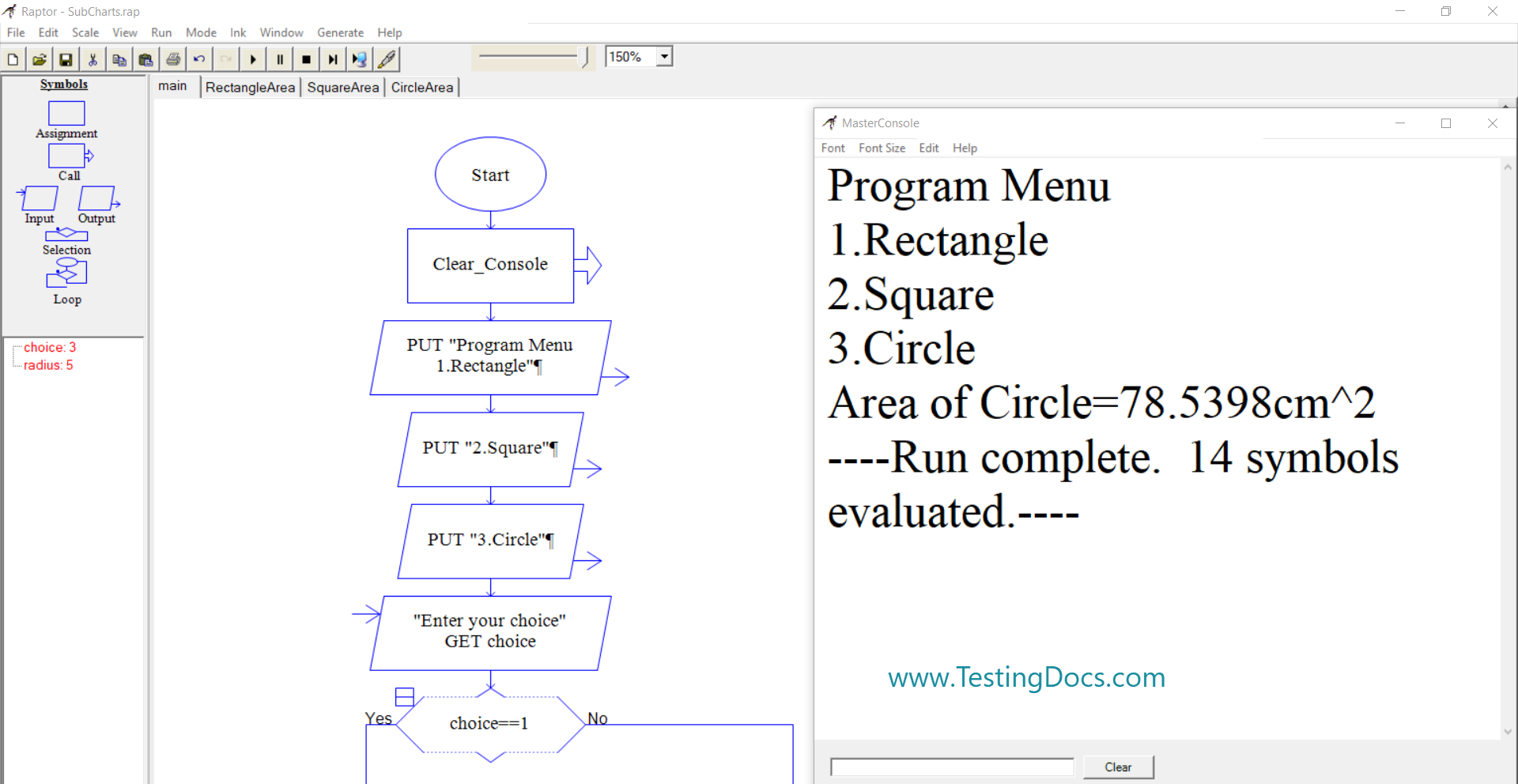# Raptor Subcharts Demo Flowchart

### Introduction

In this tutorial, we will use the Raptor Subcharts flowchart to calculate the area of different shapes. The main chart of the Demo flowchart will prompt a program menu with options.

###The main flowchart has three options for Rectangle, Square, and Circle.

Program Menu

1.Rectangle
2.Square
3.Circle

Based on the user choice we will invoke the corresponding sub chart as per the user choice.

### Rectangle Subchart

The rectangle sub chart will calculate the area of the rectangle if the user chooses the Rectangle option in the main chart.Similarly for the other two options i.e for Square and Circle, we can design the sub-charts.

### Flowchart output

Program Menu

1.Rectangle
2.Square
3.Circle
Area of Circle=78.5398cm^2
—-Run complete. 14 symbols evaluated.—-